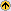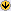## Search Results1 results returned.
To copy the URL of a document, Right Click on the document title, select "Copy Shortcut/Copy Link", then paste as needed. Only documents available to the public have this feature enabled.
TitlePrimary
AuthorAbstract / Details
View
Hard
copy
Pictures of Linear Models Bergerud, W.A.
2008
 A D
 Abstract: This handbook explains various types of statistical models, using simple graphs and simple arithmetic. Most statistical tests used in forestry research are based on the linear models of simple and multiple regression, and analyses of variance and covariance. A linear model is a weighted sum of explanatory variables used to predict or model the response variable. The weights are called parameters and since they are usually unknown, they must be estimated from the data. The differences between the observed values and those fitted by a model are minimized through (ordinary) least squares (OLS or LS) methods. This means that a type of model is chosen for the data by the researcher and then OLS chooses the specific values of the model parameters, such as the intercept and slope for a simple linear regression. The values chosen are those for which the sum of squares of the differences (SSR) between the actual and fitted values is the least. Hence the term "least squares". Several different models are usually fitted to a set of data. Pairs of models are compared where the simpler one is missing one or more parameters of the other. For instance, the mean is a simpler model than a simple linear regression model since the mean has only one parameter (namely the mean) while the regression has two (the intercept and slope) . If certain assumptions about the data are reasonable, statistical tests can be used to compare such models.
 Bergerud, W.A.. 2008. Pictures of Linear Models. British Columbia Ministry of Forests, Lands, Natural Resource Operations and Rural Development. Biometrics Information Handbook. BIO1 Topic: FLNRORD Research Program Keywords: Biometrics ISSN: Scientific Name: ISBN: English Name: Other Identifier:
To copy the URL of a document, Right Click on the document title, select "Copy Shortcut/Copy Link", then paste as needed. Only documents available to the public have this feature enabled.